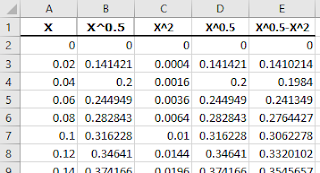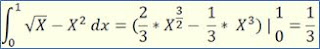## 05 August, 2021

### How to highlight space between two plotted curves / lines

If you want to enhance your Excel charts, to fill with color some target range of the data, this procedure may be helpful.

I'm providing here two examples of such enhanced charts. In addition, I've included procedure for calculation of the surface area bounded by the two curves/lines.

Chart #1

To achieve similar effects you need to follow these basic steps:

1. Start with producing data for the chart series. I've selected the two lines:  Y = 1- X^2  and   Y = X-0.5  for the chart and prepared data table consisting of five columns, like this one:

Column E lists differences between values of Columns B and C.

2. Select the series for both curves (Columns A to C) and insert the scatter chart with smooth lines on your worksheet.

3. Right-click on the chart and select all the data (five Columns) and add the third line based on data in Column E.

4. Select the line representing Column C data. Right-click and select option "Change Series Chart Type..." to the Combo Stacked Area type. Do the same with the curve representing  Column E data.

5. Select the curve representing Column B data, and click in the ribbon menu Format > Shape Fill > No Fill .

6. Do all the formatting, as you need to, related to the axes, gridlines, chart title, legend, labels, chart area, plot area, etc.

7. Using your algebraic knowledge, calculate coordinates of intersection points for the curves. In this specific case the coordinates are:

• X1 = -1.82288    Y1 = 2.32288
• X2 =  0.82288    Y2 = 0.32288

8. Knowing the coordinates you can calculate e.g. the surface area enveloped between the two curves/lines using the following integral function:

 Chart #2This chart is based on two curves:  Y = X^0.5  and   Y = X^2  and the chart data table, like this one:The coordinates of intersection points for the curves are in this case as follows:X1 = 1    Y1 = 1X2 = 0    Y2 = 0And the surface area enveloped between the two curves is calculated using the following integral function: